## What is the polar form of Negative 9 StartRoot 3 EndRoot + 9 i?

Question

What is the polar form of Negative 9 StartRoot 3 EndRoot + 9 i?

in progress 0
3 weeks 2021-08-31T12:25:21+00:00 1 Answers 0 views 0

Step-by-step explanation:

In the rectangular complex number -9√3 + 9i, which has a standard form a + bi, the a = -9√3 and the b = 9. We need this in polar form (r, θ) where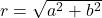and filling in: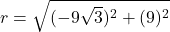(notice we do not put the i in there with the 9).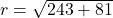so

r = 18. Now let’s move on to the angle, which is a little more difficult. The angle is found in the inverse tangent ratio: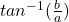so filling that in, we have: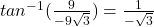Since tangent is the side opposite over the side adjacent, y is positive and x is negative in the second quadrant. This is a 30 degree angle in QII, which has a reference angle of 150 degrees. This angle in radians is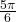, so the polar form of that number is (18,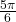)